# Excel WEEKDAY function: get day of week, weekends and workdays

If you are looking for an Excel function to get day of week from date, you've landed on the right page. This tutorial will teach you how to use the WEEKDAY formula in Excel to convert a date to a weekday name, filter, highlight and count weekends or workdays, and more.

There are a variety of functions to work with dates in Excel. The day of week function (WEEKDAY) is particularly useful for planning and scheduling, for example to determine the timeframe of a project and automatically remove weekends from the total. So, let's run through the examples one-at-a-time and see how they can help you cope with various date-related tasks in Excel.

## WEEKDAY - Excel function for day of week

The Excel WEEKDAY function is used to return the day of the week from a given date.

The result is an integer, ranging from 1 (Sunday) to 7 (Saturday) by default. If your business logic requires a different enumeration, you can configure the formula to start counting with any other day of week.

The WEEKDAY function is available in all versions of Excel 365 through 2000.

The syntax of the WEEKDAY function is as follows:

WEEKDAY(serial_number, [return_type])

Where:

Serial_number (required) - the date that you want to convert to the weekday number. It can be supplied as a serial number representing the date, as a text string in the format that Excel understands, as a reference to the cell containing the date, or by using the DATE function.

Return_type (optional) - determines what day of the week to use as the first day. If omitted, defaults to the Sun-Sat week.

Here is a list of all supported return_type values:

Return_type Number returned
1 or omitted From 1 (Sunday) to 7 (Saturday)
2 From 1 (Monday) to 7 (Sunday)
3 From 0 (Monday) to 6 (Sunday)
11 From 1 (Monday) to 7 (Sunday)
12 From 1 (Tuesday) to 7 (Monday)
13 From 1 (Wednesday) to 7 (Tuesday)
14 From 1 (Thursday) to 7 (Wednesday)
15 From 1 (Friday) to 7 (Thursday)
16 From 1 (Saturday) to 7 (Friday)
17 From 1 (Sunday) to 7 (Saturday)

Note. The return_type values 11 through 17 were introduced in Excel 2010 and therefore they cannot be used in earlier versions.

## Basic WEEKDAY formula in Excel

For starters, let's see how to use the WEEKDAY formula in its simplest form to get the day number from date.

For example, to get the weekday from date in C4 with the default Sunday - Saturday week, the formula is:

`=WEEKDAY(C4)`

If you have a serial number representing the date (e.g. brought by the DATEVALUE function), you can enter that number directly in the formula:

`=WEEKDAY(45658)`

Also, you can type the date as a text string enclosed in quotation marks directly in the formula. Just be sure to use the date format that Excel expects and can interpret:

`=WEEKDAY("1/1/2025")`

Or, supply the source date in a 100% reliable way using the DATE function:

`=WEEKDAY(DATE(2025, 1,1))`

To use the day mapping other than the default Sun-Sat, enter an appropriate number in the second argument. For example, to start counting days from Monday, the formula is:

`=WEEKDAY(C4, 2)`

In the image below, all the formulas return the day of the week corresponding to January 1, 2025, which is stored as the number 45658 internally in Excel. Depending on the value set in the second argument, the formulas output different results.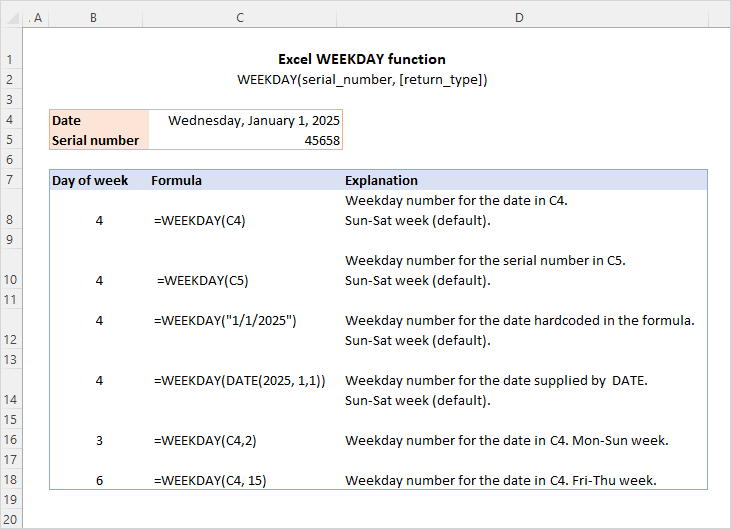At first sight, it may seem that the numbers returned by the WEEKDAY function have very little practical sense. But let's look at it from a different angle and discuss some formulas that solve real-life tasks.

## How to convert Excel date to weekday name

By design, the Excel WEEKDAY function returns the day of the week as a number. To turn the weekday number into the day name, employ the TEXT function.

To get full day names, use the "dddd" format code:

TEXT(WEEKDAY(date), "dddd")

To return abbreviated day names, the format code is "ddd":

TEXT(WEEKDAY(date), "ddd")

For example, to convert the date in A3 to the weekday name, the formula is:

`=TEXT(WEEKDAY(A3), "dddd")`

Or

`=TEXT(WEEKDAY(A3), "ddd")`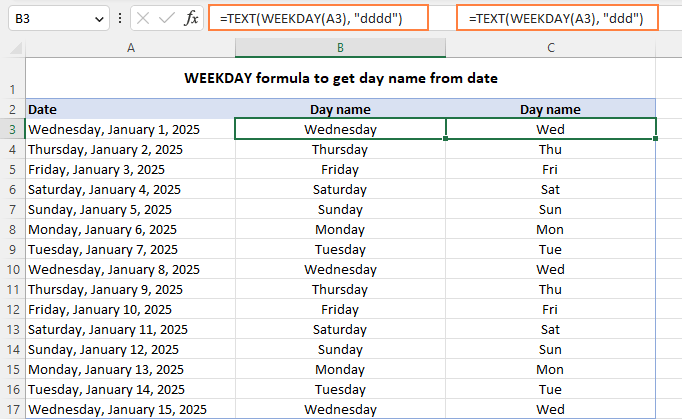Another possible solution is using WEEKDAY together with the CHOOSE function.

For example, to get an abbreviated weekday name from the date in A3, the formula goes as follows:

`=CHOOSE(WEEKDAY(A3),"Sun","Mon","Tus","Wed","Thu","Fri","Sat")`

Here, WEEKDAY returns a serial number from 1 (Sun) to 7 (Sat) and CHOOSE selects the corresponding value from the list. Since the date in A3 (Wednesday) corresponds to 4, CHOOSE outputs "Wed", which is the 4th value in the list.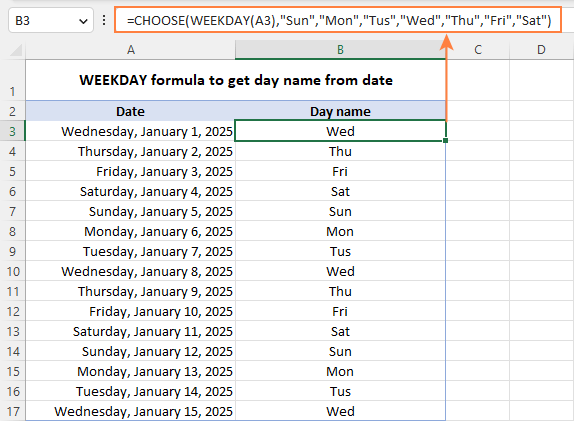Though the CHOOSE formula is slightly more cumbersome to configure, it provides more flexibility letting you output the day names in any format you want. In the above example, we show the abbreviated day names. Instead, you can deliver full names, custom abbreviations or even day names in a different language.

Tip. Another easy way to convert a date to the weekday name is by applying a custom date format. For example, the code format "dddd, mmmm d, yyyy" will have the date displayed as "Friday, January 3, 2025" while "dddd" will show just "Friday".

## Excel WEEKDAY formula to find and filter workdays and weekends

When dealing with a long list of dates, you may want to know which ones are working days and which are weekends.

To identify weekends and weekdays in Excel, build an IF statement with the nested WEEKDAY function. For example:

`=IF(WEEKDAY(A3, 2)<6, "Workday", "Weekend")`

This formula goes to cell A3 and is copied down across as many cells as needed.

In the WEEKDAY formula, you set return_type to 2, which corresponds to the Mon-Sun week where Monday is day 1. So, if the weekday number is less than 6 (Monday through Friday), the formula returns "Workday", otherwise - "Weekend".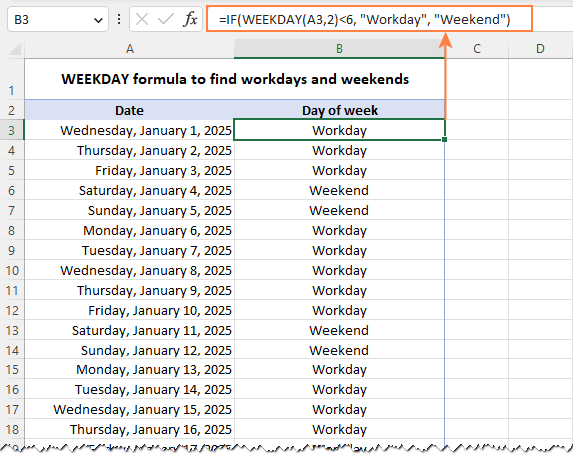To filter weekends or workdays, apply Excel filter to your dataset (Data tab > Filter) and select either "Weekend" or "Workday".

In the screenshot below, we have weekdays filtered out, so only weekends are visible: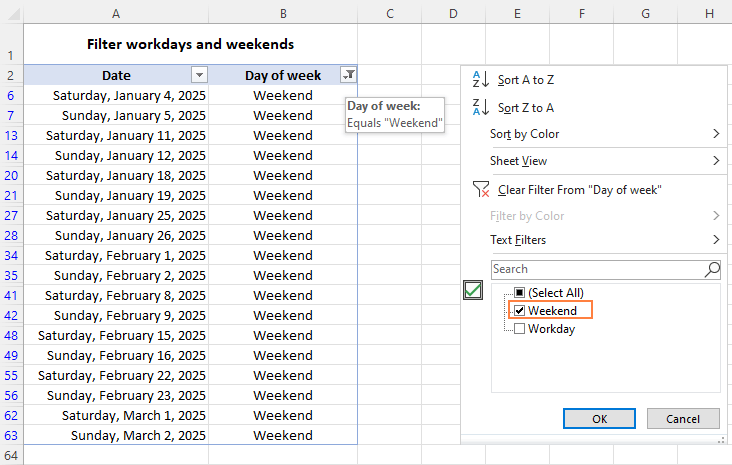If some regional office of your organization works on a different schedule where the days of rest are other than Saturday and Sunday, you can easily adjust the WEEKDAY formula to your needs by specifying a different return_type.

For example, to treat Saturday and Monday as weekends, set return_type to 12, so you'll get the "Tuesday (1) to Monday (7)" week type:

`=IF(WEEKDAY(A2, 12)<6, "Workday", "Weekend")`

## How to highlight weekends workdays and in Excel

To spot weekends and workdays in your worksheet at a glance, you can get them automatically shaded in different colors. For this, use the weekday/weekend formula discussed in the previous example with Excel conditional formatting. As the condition is implied, we only need the core WEEKDAY function without the IF wrapper.

To highlight weekends (Saturday and Sunday):

`=WEEKDAY(\$A2, 2)<6`

To highlight workdays (Monday - Friday):

`=WEEKDAY(\$A2, 2)>5`

Where A2 is the upper-left cell of the selected range.

To set up the conditional formatting rule, the steps are:

1. Select the list of dates (A2:A15 in our case).
2. On the Home tab, in the Styles group, click Conditional formatting > New Rule.
3. In the New Formatting Rule dialog box, select Use a formula to determine which cells to format.
4. In the Format values where this formula is true box, enter the above-mentioned formula for weekends or weekdays.
5. Click the Format button and select the desired format.
6. Click OK twice to save the changes and close the dialog windows.

For the detailed information on each step, please see How to set up conditional formatting with formula.

The result looks pretty nice, doesn't it?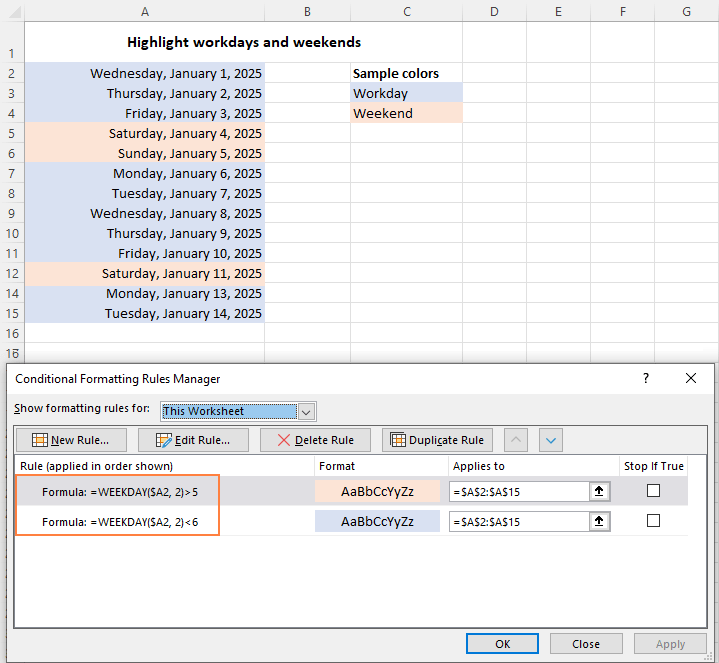## How to count weekdays and weekends in Excel

To get the number of weekdays or weekends in the list of dates, you can use the WEEKDAY function in combination with SUM. For example:

To count weekends, the formula in D3 is:

`=SUM(--(WEEKDAY(A3:A20, 2)>5))`

To count weekdays, the formula in D4 takes this form:

`=SUM(--(WEEKDAY(A3:A20, 2)<6))`

In Excel 365 and Excel 2021 that handle arrays natively, this works as a regular formula as shown in the screenshot below. In Excel 2019 and earlier, press Ctrl + Shift + Enter to make it an array formula.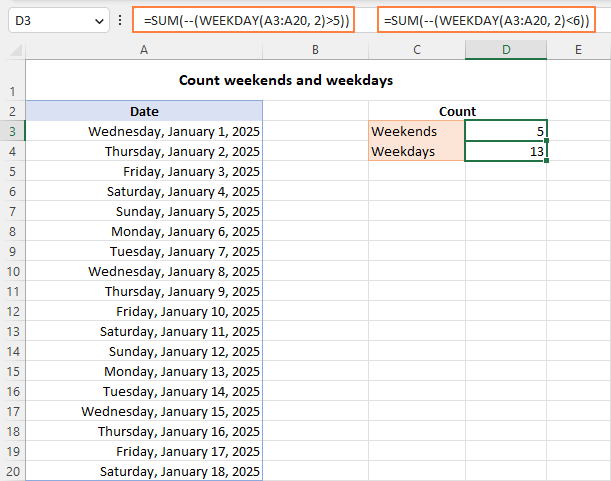How these formulas work:

The WEEKDAY function with return_type set to 2 returns a day number from 1 (Mon) to 7 (Sun) for each date in the range A3:A20. The logical expression checks if the returned numbers are greater than 5 (for weekends) or less than 6 (for weekdays). The result of this operation is an array of TRUE and FALSE values.

The double negation (--) coerces the logical values to 1's and 0's. And the SUM function adds them up. Given that 1 (TRUE) represents the days to be counted and 0 (FALSE) the days to be ignored, you get the desired result.

Tip. To calculate weekdays between two dates, use the NETWORKDAYS or NETWORKDAYS.INTL function.

## If weekday then, if Saturday or Sunday then

Finally, let's discuss a bit more specific case that shows how to determine the day of the week, and if it's Saturday or Sunday then do something, if a weekday then do something else.

IF(WEEKDAY(cell, 2)>5, if_weekend_then, if_weekday_then)

Suppose you are calculating payments for employees who have done some extra work on their days off, so you need to apply different payments rates for workdays and weekends. This can be done using the following IF statement:

• In the logical_test argument, nest the WEEKDAY function that checks whether a given day is a workday or weekend.
• In the value_if_true argument, multiply the number of working hours by the weekend rate (G4).
• In the value_if_false argument, multiply the number of working hours by the workday rate (G3).

The complete formula in D3 takes this form:

`=IF(WEEKDAY(B3, 2)>5, C3*\$G\$4, C3*\$G\$3)`

For the formula to copy correctly to the below cells, be sure to lock the rate cell addresses with the \$ sign (like \$G\$4).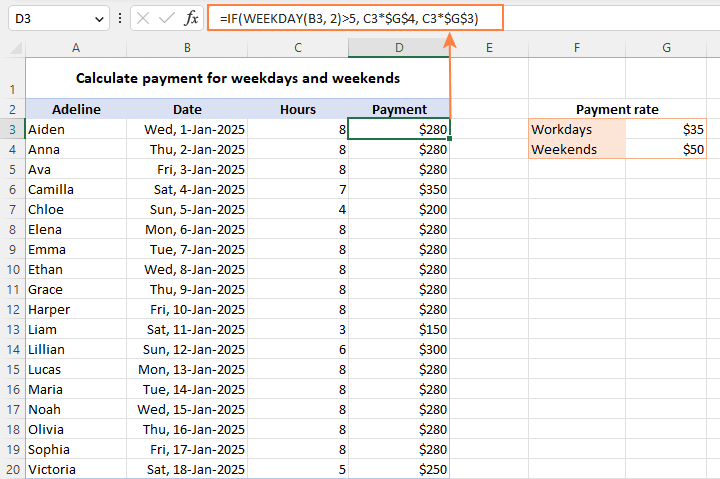## WEEKDAY function not working

Generally, there are two common errors that a WEEKDAY formula may return:

#VALUE! error occurs if either:

• Serial_number or return_type is non-numeric.
• Serial_number is out of supported dates range (1900 to 9999).

#NUM! error occurs when return_type is out of the permitted range (1-3 or 11-17).

This is how to use the WEEKDAY function in Excel to manipulate days of week. In the next article, we will explore Excel functions to operate on bigger time units such as weeks, months and years. Please stay tuned and thank you for reading!

WEEKDAY formula in Excel - examples (.xlsx file)

## You may also be interested in

1. Can anyone help with what is wrong in this formula
=COUNTIFS(B2:B5,">=EOMONTH(TODAY(),-2) +1",C2:C5,"N/A",D2:D5,"<=EOMONTH(TODAY(),-2) +28")
here is the data:
B2:B5 =
01/02/2022
01/01/2022
01/02/2022
01/01/2022
C2:C5 =
04/09/2022
N/A
30/03/2022
N/A
D2:D5 =
28/02/2022
31/01/2022
03/03/2022
30/01/2022

• Hello!
Your formula contains errors. Read carefully how to use COUNTIFS function with dates.

=COUNTIFS(B2:B5,">="&(EOMONTH(TODAY(),-2)+1), C2:C5, "N/A", D2:D5,"<="&(EOMONTH(TODAY(),-2)+28))

The formula returns 0 because all dates in column D are greater than 28-Jan-22

2. How do i formulate it to say yes if it is a weekday and no if it is a weekend?

• Hello!
Use the WEEKDAY function to determine the day of the week. Then use that in your IF formula.

=IF(WEEKDAY(TODAY())>5,"Yes","No")

3. Hi! Given a column of dates in the form, month, followed by the year...such as...
Jan-2000
Feb-2000
Mar-2000
.
.
.
December-2000

How can I separate the month from the year? I would like the months in one column, followed by its year in the next column. The goal is to create fields or "month" and "year" in a pivot table. Thank you.

Sue

4. Greetings from Ireland, is it possible to configure the WEEKNUM formula so that it yields a day of the Mon-Fri works well? For example, Monday would be "XX.1", Tuesday would be "XX.2", etc.?

• Hello!
I don't really understand what you want to calculate.
However, I’ll try to guess and offer you the following formula:

=WEEKNUM(A4)&"."&WEEKDAY(A4,2)

If this is not what you wanted, please describe the problem in more detail.

5. Hey, I was wondering if you could possibly help me.

I am trying to figure out the amount of days late a tenants rent is. I am using this formula but it is not working, am I doing it wrong?

=DAYS((today(),datevalue(2021/06/08))

Basically, I just need to know if the tenant's rent is due on the 8th of any given month (for this we can say June 8 2021) and by today the rent still is not paid how many days late are they, including today. I mean I can do the math and I know it would be 178 days late, but is there a formula to put in the cell so it will calculate it automatically? Also, I think that if I just change the month in the following cells (ex July 8, 2021, Aug 8 2021) it would still work? Yes?

Thank you in advance, any help you can offer would be super. I think if I were to use such a formula it would be super useful when I am sending the tenant reminder letters that their rent is late, so if they needed the file they could make a spreadsheet of their own if they wanted to.

(Just because I feel it is important to add: No, I am not heartless, I am not trying to put tenants out on the streets, but looking to give them a breakdown of their rent so they can apply for help paying their back rent due to the current pandemic affecting them, if that is what they need.)

• Hello!
In the DATEVALUE function, the argument must be written as text. Add quotation marks.

datevalue("2021/06/08")

You can also calculate the difference between dates using the DATEDIF function.

• Thank you so much, That is Perfect! it works now. I appreciate you.

6. Hi,
I Has One Problem In Google Sheet.
Why My Google Sheet Only Cell G29 & G30 Cannot Be Change To "ddd"?
I'm Confuse.

7. Hi,
I want to mentioned 0 on saturday or sunday of every month on rest date & weekdays reflects 1. how to do this in execl,

8. Hi,
I have data in column A as below
Mon
Tue
Wed
Thu
Fri
Tue .... and so on depending upon the working day of a person in shift.
So practically speaking the working day of the next week is Tue and not Mon.
How do i post in Column B that start of week day for that person.
Thanks and regards
Govind

9. Hi, I've spend many weeks on trying to find a solution to calculate number of days per week. My Sheet has twelve columns for each month of the year. Below each month I have 5 columns for W1, W2, W3, W4 and Week 5. I need to know number of days for week1, meaning from 1 Jan till first Friday. Then Week2 till second Friday (will always be 5 days) and so on for all weeks, except last week will be from last Monday of the month till the end of the month. Currently I do this manually for all 52 weeks of the year by looking at calendar.

10. Hi ther, plz help me on this,

I have to do fortnightly payments (on Every alternate Friday) if i received a job card on monday or Thursday, that payment date( due date) should be reflect as alternative friday date on received date in excel payment sheet.

11. Svetlana, Merry me!

12. Dear Sir,
My doubt is quite a basic one. I have the Seven Days of the week. I want to now create a dummy for those days as

Monday to Thursday = Weekday
Friday to Sunday = Weekend

How do I do that in Excel?

• Hello!
Use the WORKDAY.INTL and NETWORKDAYS.INTL functions.
I hope this will help.

13. Hello

I want to identify last Thursday of each month from all Thursdays by a formula from given dates of style 04FEB2021,11FEB2021, and so on. Thanking you in advance.

• Hello!
To find the last Thursday of the month use this formula

=EOMONTH(\$A2,0)-MOD(EOMONTH(\$A2,0) +2,7)

A2 - cell with date.

14. Hello,

I have a very complex tracking sheet for my work.

One of the functions counts the amounts of any specified day within a date range.

I would like to add one more part to this formula and am not sure how.

Current formula:

=SUMPRODUCT(--(WEEKDAY(ROW(INDIRECT(Z2&":"&AA2)))=V2))

Is there a way so that my formula will equal 9, (instead of 10) in cell AC when it is complete? The formula would need to use a list of populated holidays from another sheet titled “Lists”. That range of holidays is - F2:F5. When it calculates, it will determine that because the Day after thanksgiving is a Friday and falls within that range, it will automatically subtract an additional day.

How can this be accomplished?

• Hello!
Unfortunately, without seeing your data it is impossible to give you advice.

I'm sorry, it is not very clear what result you want to get. Could you please describe your task in more detail and send us a small sample workbook with the source data and expected result to support@ablebits.com? Please shorten your tables to 10-20 rows/columns and include the link to your blog comment.

Thank you!! LIFE SAVER!!

15. I have a production schedule on excel that the date column feeds off another cell. Basically todays date , plus the amount of time in the daily work schedule. Formula currently is = I3 +TODAY() So if today is 8/28 and I have 20 + jobs in lines I3,I4,........and so on. Each one adds up to .25 of a 8 hr shift. So my sheet shows 8/28 for the 1st 4 lines, on the 5th line it turns to 8/29 as it should as my 8 hr capacity is used up. My issue is I cant figure out how to take out Sat. and Sun and holidays from being populated as dates, therefore making it look like I can complete jobs sooner cause its using those 2 day we dont work on.

• Hello!
If I understand your task correctly, the following formula should work for you:

=WORKDAY(TODAY(),I3,E1:E6)

16. I need to generate a formula to where If Tuesday a cell value = 1 and if any other day of the week the cell value = 2.
I have been trying to work with the =Weekday but so far, unable to get it to work.
Thanks for any advice in working through this.

Thanks
John

17. sir i need formula for create a list of working days dates ignore Saturday,Sunday dates require date list based on date in a cell reference (like 7/1/2020,7/2/2020,7/3/2020.7/6/2020,7/7/2020) thank you

• Hello!
I recommend reading this article on calculating working days. If there are difficulties, give an example of the source data and the expected result.
It’ll help me understand it better and find a solution for you.

18. I have a cell with a date that is changed each week in A1 and formula to give me the day of week starting with Sunday ending Saturday and also give the date of each day based on the date in cell A1. The following formula is used in each cell C5:I5 =DATE(YEAR(\$A\$1),MONTH(\$A\$1),DAY(\$A\$1)) C5 is Sunday Then D5:I5 adjust the Day(\$A\$1+1) it's +1 for Monday,+2 for Tuesday,+3 for Wednesday,+4 for Thursday,+5 for Friday,+6 Saturday. My problem is since June only has 30 days it skewed the DAYS of the week but the DATE is correct, so it reads Sunday 28th Monday 29th Tuesday 30th Monday 1st Thursday 2nd Wednesday 3rd Thursday 4th. If I change the formula starting on the first thru 4th to be =DATE(YEAR(\$A\$1),MONTH(\$A\$1+6),DAY(I5+1)) then it works until there is a change from 30 to 31 day month.

Is there a formula that will recognize and make the adjustment automatically?

• Hello Cindy!
I propose the formula = \$ A \$ 1 in cell C5, the formula = \$ A \$ 1 + 1 in cell D5, the formula = \$ A \$ 1 + 2 in cell E5, and so on. I think this will help solve the problem.

19. Hi, please would you know the function or a way i can use to get the weekend (Saturday) date of a particular day in a week. Let's say i want to automatically get the weekend date of today (6/23/2020) in my cell. conditional formatting didn't work for me. Thanks in anticipation.

• Hello Michael!
If I understand your task correctly, the following formula should work for you:

=A1+(8-WEEKDAY(A1,16))

In WEEKDAY function, use parameter 16
I hope this will help

• Wooooooow! I wish could give a hug right now. It worked perfectly. You saved me a lot of stress. Thanks a lot, great work you're doing here!

• Hello!
The formula below will do the trick for you:

=WEEKDAY(B3,2) > 5

• Thank you..i think there is something wrong with my excel...all the other formulas are working fine,, but only this weekend highlighting formula is not working for me.

21. Hello
Can you help me?
I need to add 2 days if the date falls on a Saturday and if it falls on sundays, we add 1 day

• Hello!
You need to determine the day of the week using the WEEKDAY function and apply it as a condition in the IF function

=IF(WEEKDAY(A7,2)=7, A7+1, IF(WEEKDAY(A7,2)=6, A7+2,A7))

Hope this is what you need.

22. Hi, I am having an issue and I do not understand why this is happening.
My year begins on a Sunday, December 29th (cell B1) and ends on Saturday, January 4th (cell B4)
Since the formula WEEKNUM refers to the date in cell B1, and is a Sunday, I omitted the type since the week begins on a Sunday, per Excel reference sheet: -> System 1: The week containing January 1 is the first week of the year, and is numbered week 1.

But whatever I do, it always returns as week number 53 instead of 1, as it should be.

The second issue is that the second week begins on Sunday, January 5th (cell J1) and ends on Saturday, January 11th (cell P1). Since the formula still refers to the Sunday of the first day of this week (January 5th), It returns as week number 2.
That would be correct week number is the first week wasn't considered as week #1.
Because of this issue, Week 1 does not exist and causes problems with futur weeks and years.

I would be very grateful if you could help me find a solution to my problem.
Thank you very much and have a good day :)

• Sorry, I meant to say in my second sentence: "I am using Excel 2013, I got a bit distracted"

23. Hello,
I have a question and maybe someone could help me here.
How can I make a formula to count for the whole year weeks and dates with more then 1 cell with the same date?
FX:
Week1 01/01/2020
Week1 01/01/2020
week1 02/01/2020
week1 02/01/2020

Thank you in advance and have a nice day!

• Hello!
For me to be able to help you better, please describe your task in more detail. Please let me know in more detail what you were trying to find, what formula you used and what problem or error occurred. Give an example of the source data and the expected result. For example, Week1 01/01/2020 - is it one cell or several?
It’ll help me understand it better and find a solution for you. Thank you.

24. Hello,

I am new to excel. Could you please help me? Is it possible to set a range by using "TODAY()" with a certain cell? For example: =SUMPRODUCT(--(WEEKDAY(TODAY():B34)={3,5}))

• Hello!
In the WEEKDAY function, you can record WEEKDAY(B33:B34). And in cell B33 write the formula =TODAY(). For me to be able to help you better, please describe your task in more detail. Please let me know in more detail what you were trying to find, what problem or error occurred. It’ll help me understand it better and find a solution for you. Thank you.

• I have a table of lunch expenses. I have dates of May on B4:B34, the expenses on E4:E34, and a fixed budget for the month. I need to sum all lunch expenses except Tuesdays and Thursdays and figure out the left budget for lunch expense to spend till the end of the month. So What I want is to sum all Tuesdays and Thursdays from today to the end of the month so every morning whenever I open the file I would know how much is left to spend for lunch. That formula is just a part portion. Sorry, if I didn't make it clear still.

• Hello!
To add all expenses for Tuesdays and Thursdays, use the formula

=SUMPRODUCT((WEEKDAY(B4:B34)=3) + (WEEKDAY(B4:B34)=5),E4:E34)

I hope this will help, otherwise please do not hesitate to contact me anytime.

• Hello,
Thanks for taking time to help me out. What I want to do though is to use "today()" within sumproduct fuction. I want the computer automatically calculate left days of the month from "today" excluding Tuesdays and Thursdays, not the amount of the expenses but the actually days.

I need the mathematical number sentence like this:
Lunch Budget ÷ (Days Left - (Tuesdays & Thursdays from the left days)*)
* Since I have to check the left lunch budget each day I want to use "today()" function.

I came up with this formula and it worked:
P27(LUNCH BUDGET) | B34(LAST DAY OF THE MONTH) | B31(TODAY'S DATE)

P27 / ((NETWORKDAYS(TODAY(),B34)) - SUMPRODUCT(--(WEEKDAY(B31:B34)={3,5})))

but, I want to make it like this:
P27 / ((NETWORKDAYS(TODAY(),B34)) - SUMPRODUCT(--(WEEKDAY(today():B34)={3,5}))) or
P27 / ((NETWORKDAYS(TODAY(),B34)) - SUMPRODUCT(--(NETWORKDAYS(today(),B34)={3,5})))
but both won't work.

I hope it help you to understand better as to what I want to figure out.

25. Hi,
I try to get a result for the following example: if today is 04/14/2020, and last month 03/14/2020 fell on the weekend, what formula does it apply to bring me the next working day in March = 03/16/2020?
Thx

• Hello Cristian!
If I understand your task correctly, maybe the following formula should work for you:

=IF(WEEKDAY(EDATE(A1,-1))>5, WORKDAY(EDATE(A1,-1)-1,1), EDATE(A1,-1))

Hope you’ll find this information helpful.

26. In weeknum suggestions why we have to use different numbers for monday like 2,3(0-6),11 and for sunday 1,17. For ex: for sunday we can use 1 right, why 17 also there for sunday, and where will have to use 17, is there any logic for using 1 or 17, like here we have to use only 17 for sunday but not 1, please let me know this, thankyou

• Hello Kiwi!
The list of possible values for the return_type argument in the WEEKDAY function is set by Microsoft. You simply choose a type from the list which suits better for your task. You can use any of the them as they just determine what day of the week to use as the first day.

27. Hi, I am trying something a bit different than what ever everyone else seems to be doing. I hope you can help me as I've been trying different ways for a very long time now and am getting nowhere fast.
I am building a weekly schedule and can allocate weekdays easily. I am trying to enter holidays into this formula but it does not work. I don't care about how many days it takes. I am only concerned with what my end date will be (without weekends or holidays).
This is what I've been entering so far..Example (=weekday,1). "weekday" being in the box next to it. then I simply follow through with that formula across the row and it self tabulates the weekdays only. When I try to enter a holiday with it...example (=weekday,1, B6:B12) "these would be dates in a different box", it does nothing. hope this makes sense. Please help if you can.

• Hello Rob!
If I understand your task correctly, the following formula should work for you:
=IF(AND(WEEKDAY(A1,2)<6,ISNA(VLOOKUP(A1,\$G\$1:\$G\$7,1,FALSE))),"workday","holiday")
where \$G\$1:\$G\$7 - list of holidays.

28. 10-1-2019 1:30AM, - 12-1-2020 3:10AM total year,munth,dd, Hh,mm Excel convert plg sent

29. i have this date 08-01-10 11:15
and i want to transform it to day of the week...
any suggestions?

30. Day 1 in Excel is January 1, 1900.
Weekday of this date gives a Sunday.
However, when you Google for a calendar image of January 1900 you get a Monday.
Can anyone explain this anomaly?
Thanks,
Fred

31. I have a range of random dates in column b3:b30 and wish to find the oldest date and convert to the corresponding Monday

How do I nest and use the MIN and Weekday function in excel?

32. I have problem when creating =WEEKDAY(\$A2,2)>5 for year 2020 Feb 28 cross over to Mar 01. My "Sat" did not appear. It turns out to be a "Sun". I've checked the cell, the date is 01/03/2020 but ddd turn out to be a "Sun". How can I solve this?

33. I am using conditional formatting to highlight weekends. If the first falls on a weekend it does not highlight. I am using =OR(WEEKDAY(\$A1)=7,WEEKDAY(\$A1)=1). What is wrong?

• Hello Bill,
Since 30 and 31 of December is the 53rd week of 2019 and 1st to 5th January is the first week of 2020, the formula for this particular week needs some modification. Please try the one below:

=IF(OR(WEEKNUM(\$A1, 1) = 53,WEEKNUM(\$A1, 1) = 1), TRUE)

Hope it'll work for you.

• Thanks for the tip, but it didn't quite work. I am using a new spreadsheet for the year. However, I did get it to work by starting it in the correct cell (not \$A1 but \$A2). I like your site, I have learned a lot from it.

• Thank you for replying, Bill. Glad to hear you found a solution!

34. Sir..i need to formula.one cell i enter month and i get result in column sequences ..wed..thu..sun..wed..thu..sun..wed..thu with date for this month

35. Can I actually do this? :)
I have this format in the cells for date and time:
04:35 PM EDT Oct 29 2019
How can I create a formula for finding how many minutes elapsed between cells in column A (arrival time) and column B (departure time)?
I want to calculate how long my visitors spent in my office.

36. Hi Team,
I am working in a BPO industry. I need to track the production as well as the other details in day wise. For example The date is 1st November, in need to track the Target, Achieved, Production. So I have merged the 1st November header has been merged into three above cells. I do not have any issues on this. Please help me out to convert into weekly basis with the same header.

37. I'm looking to calculate the date of the year based on a day.
I'm doing some analysis using 31 years of day and have a mean value, 45 (i.e. day 45 of 365). I want to convert 45 to represent a date, so I don't have a generic year to plug in.
What would be the excel formula to do this?

38. How can i calculate the first (1st) day of each calendar month or the immediately preceding Business Day if the first (1st) day of such calendar month is not a Business Day?

39. Is there a way to calculate the day of the week with the numbering 1-14 instead of 1-7?

40. hi,
i need to calculate age between 2 dates excluding weekends?

41. Hi, I need to return a custom day (i.e S for Sunday and so forth).. I used the CHOOSE and WEEKDAy function but then the formula doesn't drag across and then follow the next day sequence. It's just copying the S from the cell before. How do I get this formula to work across the schedule I am creating? =CHOOSE(WEEKDAY(cell reference), "S", "M", "T", etc etc. Can you please help as I will using this to setup monthly work schedules

43. Hello . I'm following all the functions from last week. You are Awesome because all your examples are very useful..

Question : For ex : I have a one date as a reference and from this reference date i need to get 3 different dates i.e ( 7 days before the ref date, 2 days before the ref date & 1 day before the ref date) but the twist is that the new date should fall on Weekday and not on Weekend( i.e Sat & sun).

44. I am working on something and I need to enter a formula that counts a large number of days of the week that automatically adds up how many for example Fridays are in that list.

45. may i get the answer in Microsoft Excel to these questions and reassure me if I can be selected. 1.sunday - Saturday + 4 Monday =? , Thursday - 3 Saturday + monthly total = 2. Summation = Sunday of the month divided by summation Monday of the month. 3. 40% of the Wednesday of the month multiplying by 20% of Friday. I'm counting on you really.

46. Condition 1 should meet any text
Condition 2 if the date exceeds or equal the current date (eg: 16th of any month), it should return a value 2
Condition 3 if the date is between 1st and 15th of the same month, it should return a value of 1
Condition 4 if the date is of the preceding month, it should return a value of 0

My formula
=if(and(a1="A", or(today()>=date(2019,3,16), 2, if(a1="A", or(today()=date(2019,3,1),1, if(a1="A", or(today()<date(2019,3,1),0)))

This formula is not working . could you please let me know what is the syntax error

47. Hello, I've got a problem with a specific formula.

I have 2 cells generating dates:
Todays date (using =now () ) - In the format of dd-mm-yy hh:mm:ss
Working date (using =workday(todays date,5)+time(15,0,0) so it adds +5 to working date + declares time of 15:00:00. For my purpose, I need the format to remain dd-mm-yyyy 15:00:00.

The issue that I'm having is that once the time passes 15:30:00 on today's date (=now() function) - I need the working date to increase from 5 to 6, so it becomes tomorrow's work.

Kind Regards,
James.

• I forgot to add, this will probably need to be converted to an IF function, my cell references are:

Todays Date = I2 ( =NOW() )
Working Date = I3 ( =WORKDAY(I2,5)+TIME(15,0,0) )

48. Say I want an "IF" formula that checks a cell (a1) with a date indicated then on a different cell (b1) it validates the indicated date on cell A1 that its already pass 3 business days once confirmed cell B1 would indicate as a "Yes" if not then a "No", is this possible?

49. Dear sir i want to account the month. from start date to end date
Example

01-12-2018 - 01-06-2018 total 06 month

50. If the date field is blank how do I get the return cell day of the week to stay blank? The =WEEKDAY(A2) formula is working fine and reporting as needed into the day of the week when the date is filled in, but if the date cell is blank yet (haven't added that data line yet) how do I get the day of the week cell to stay blank also?

• Try:

=iferror(weekday(a2), " ")